# User:Trefor

(diff) ← Older revision | Latest revision (diff) | Newer revision → (diff)
Announcements go here

NOTE: This page is used as a placeholder for incomplete typed class notes.

## Typed Notes

The notes below are by the students and for the students. Hopefully they are useful, but they come with no guarantee of any kind.

We start with a one further comment on Van-Kampen:

Let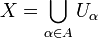$X = \bigcup_{\alpha\in A}U_{\alpha}$, with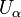$U_{\alpha}$ open and with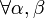$\forall \alpha,\beta$ then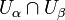$U_{\alpha}\cap U_{\beta}$ connected.

Then,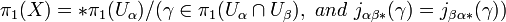$\pi_1(X) = *\pi_1(U_{\alpha})/(\gamma\in\pi_1(U_{\alpha}\cap U_{\beta}),\ and\ j_{\alpha\beta*}(\gamma) = j_{\beta\alpha*}(\gamma))$

We actually also need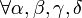$\forall\alpha,\beta,\gamma,\delta$ then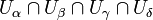$U_{\alpha}\cap U_{\beta}\cap U_{\gamma}\cap U_{\delta}$ to be connected. Recall we previously had a square grid in our homotopy, so needed the four sections around a grid point to intersect in a connected way.

However, we can employ a clever trick to reduce this to only needing triples of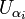$U_{\alpha_i}$ to be connected. That is, instead of covering it was squares on top of each other, cover it with rectangles arranged in the way bricks are laid in a wall.

Definition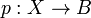$p:X\rightarrow B$ is a "covering" if, for some fixed discrete set F, every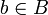$b\in B$ has a neighborhood U such that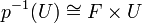$p^{-1}(U)\cong F\times U$. We have the natural map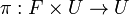$\pi:F\times U\rightarrow U$

Now a naive dream would be to classify all such covering spaces.

Example 1

The double annulus depicted above is a covering space for the single annulus

Example 2

Consider a series of rectangular planes stacked on top of each other. Make a small cut in each plane, and then glue one side of the cut to the opposite side in the plane above. Finally glue the remaining unglued sides in the very top and bottom planes to each other.

Analogously this can be done in higher dimensions.

Theorem

For a "decent" B (a topological condition to be discussed later) there is a bijection between connected coverings of B and subgroups of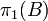$\pi_1(B)$

given by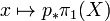$x\mapsto p_*\pi_1(X)$ included in$\pi_1(B)$

Example 3

Consider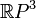$\mathbb{R}P^3$

Now,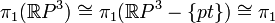$\pi_1(\mathbb{R}P^3) \cong\pi_1(\mathbb{R}P^3 - \{pt\})\cong \pi_1$(equator with identification of antipodes)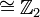$\cong\mathbb{Z}_2$ as we have previously computed.

There are only two subgroups of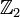$\mathbb{Z}_2$, namely {e} and$\mathbb{Z}_2$

Lets consider the case where the subgroup is$\mathbb{Z}_2$. Then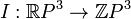$I:\mathbb{R}P^3\rightarrow\mathbb{Z}P^3$ the identity covering with F merely a point. So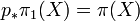$p_*\pi_1(X) = \pi(X)$

For {e},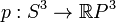$p:S^3\rightarrow\mathbb{R}P^3$ is the other covering.

Aside: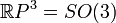$\mathbb{R}P^3 = SO(3)$

Consider a belt. A point on it can be associated with three vectors. One vector is tangent to the belt in the direction of one end. The other vector is normal to the belt. And the third is normal to both of these.

Now fix the orientation of the end points. Hence, we can think of the belt as a path in SO(3) as a homotopy. Pulling the belt tight is the identity homotopy.

We note that if one twists the orientation of the end 360 degree, then this is non trivial and there is no way to return this to the identity while holding the ends fixed in orientation. However, if you twists the orientation of an end point 720 degrees then in fact you CAN "untwist" the belt without changing the orientation of the endpoints!